Courses

# JEE Main Chemistry Mock - 1

## 25 Questions MCQ Test Mock Test Series for JEE Main & Advanced 2021 | JEE Main Chemistry Mock - 1

Description
This mock test of JEE Main Chemistry Mock - 1 for JEE helps you for every JEE entrance exam. This contains 25 Multiple Choice Questions for JEE JEE Main Chemistry Mock - 1 (mcq) to study with solutions a complete question bank. The solved questions answers in this JEE Main Chemistry Mock - 1 quiz give you a good mix of easy questions and tough questions. JEE students definitely take this JEE Main Chemistry Mock - 1 exercise for a better result in the exam. You can find other JEE Main Chemistry Mock - 1 extra questions, long questions & short questions for JEE on EduRev as well by searching above.
QUESTION: 1

### Alcohols of low molecular weight are

Solution:

Alcohols are soluble in water. This is due to the hydroxyl group in the alcohol which is able to form hydrogen bonds with water molecules. Alcohols with a smaller hydrocarbon chain are very soluble. As the length of the hydrocarbon chain increases, the solubility in water decreases.

QUESTION: 2

### Benzyl alcohol is obtained from benzaldehyde by

Solution: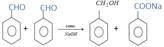QUESTION: 3

### The main structure features of proteins is

Solution:

The chemical bond formed between amino acids, constituting the primary linkagein all protein structures. In a peptide bond, the carboxyl group (COOH) of one amino acid bonds with the amino group (NH2) of another, forming the sequence CONH and releasing water (H2O).

QUESTION: 4

Chemical equilibrium is dynamic in nature, because

Solution:

A chemical equilibrium is dynamic in nature which in other words means that reactions continue to occur in both forward as well as backward direction with the same speed. As a result, the amount of product formed immediately reacts backward to give reactants and so there is no change in concentration of reactant or product with the passage of time.

QUESTION: 5

The indicator that is obtained by coupling the diazonium salt of sulphanilic acid with N,N-dimethylaniline is

Solution: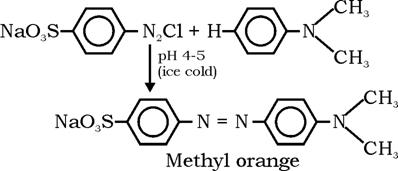QUESTION: 6

The standard e.m.f. of a galvanic cell, with standard electrode potentials of zinc equal to -0.76 V and that of copper equal to + 0.34 V, is

Solution:

Ecell = E right - E left
= E•Cu2+/Cu - E•Zn2+/Zn
= (+0.34v) - (-0.76v)
=0.34+0.76
=1.1v

QUESTION: 7
Which of the following is true for the order of a reaction?
Solution:
QUESTION: 8

When 10gram of methane is completely burnt in oxygen, the heat evolved is 560kJ. What is the heat of combustion (in kJ/mole) of methane?

Solution:

Heat of combustion of CH4 =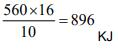QUESTION: 9

Which of the following is most electronegative?

Solution:

The electronegativity values for the representative elements decreses down a group because of the increase in atomic radius as well as screening effect. Thus, carbon has highest electronegativity in a group.

QUESTION: 10

The catalyst used for the polymerization of olefins is

Solution:

A Ziegler–Natta catalyst, named after Karl Ziegler and Giulio Natta, is a catalyst used in the synthesis of polymers of 1-alkenes (alpha-olefins).

QUESTION: 11

When one Faraday current is passed which of the following would deposit one gram atomic weight of the metal?

Solution:

Hint: For Na+, GEW=GAW

QUESTION: 12

A white salt is readily solubule in water and gives a colourless solution with a pH of about 9. The salt would be

Solution:

CH₃COONa undergoes anionic hydrolysis and gives basic solution (pH ≈ 9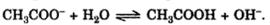QUESTION: 13

The pH of a solution of hydrochloric acid is 4. The molarity of this solution is

Solution:

The pH of a solution is equal to the base 10 logarithm of the H+ concentration, multiplied by -1. If you know the pH of a water solution, you can use this formula in reverse to find the antilogarithm and calculate the H+ concentration in that solution.

QUESTION: 14

Which of the following is least acidic?

Solution:
QUESTION: 15

Which one of the following can be considered as weak electrolyte ?

Solution:

CH3COOH does not ionise or dissociate completely and hence it is a weak electrolyte.

QUESTION: 16

Which of the following is not a Lewis acid ?

Solution:
QUESTION: 17

Which is not true about polymers?

Solution:

A polymer sample contains chains of varying lengths and therefore molecular mass is always expressed as an average.The molecular weight of polymers are very high,due to which they are expressed in two ways:-

Number average molecular mass and weight average molecular mass.

QUESTION: 18

A solid cube has CsCl type structure. If the edge length of the unit cell is 404 pm, what is the distance between A⁺ and B⁻ ions?

Solution:

In CsCl structure the distance the closest approach between the cation and anion is equal to half of the body of the diagonal of a cube.
Body diagonal = 3–√×3× Edge Length

=3–√×404

=699.74pm
The distance of the closest approach = 1/2× Body diagonal
=1/2×699.74 = 349.87 pm = 349.9pm

QUESTION: 19

Which of the following has maximum Schottky defect?

Solution:

More comparable the size of anion and cation, more is the chance of Schottky defect and Naand Cl- ions are almost comparable in size hence m ore probability to show  Schottky defect.

QUESTION: 20

Select the correct statement regarding the aromatic nitrogen molecule

Solution:

In pyrrole, the lone pair of electrons belonging to the nitrogen is part of the aromatic ring. However, in pyridine it is part of an sp2-hybridized orbital.

*Answer can only contain numeric values
QUESTION: 21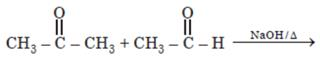Number of possible self aldol condensation product on heating with NaOH?

Solution: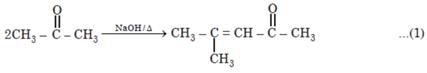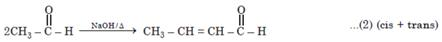*Answer can only contain numeric values
QUESTION: 22

Among the following the total number of elements which produce H2 gas with NaOH is Zn, Al, Sn, Pb, P, S

Solution:

Zn + 2NaOH → Na2ZnO2 + H
2AI + 2NaOH + H2O → 2NaAlO2 + 3H
Sn + 2NaOH + H2O → NaSnO3 + 2H2­
Pb + 2NaOH + H2O → Na2SiO3 + 2H2 ↑
S8 + 12NaOH — → 4Na2S + 4Na2S2O3 + 6H2O ↑
P4 + 3NaOH + 3H2O → PH3­↑ + 3NaH2PO2­

*Answer can only contain numeric values
QUESTION: 23

An organic compound P contains 62.07% carbon and 10.34% hydrogen and rest oxygen. Its vapour density is 29. This compound does not react with sodium metal, but its 2.9 g combines with X g of bromine (to give dibromo addition product). Find out value of (Y–X). (Where Y is total number of possible isomers of given organic compound P)
[Atomic mass : Br = 80]

Solution:

Molecular formula from calculation comes to be C3H6O i.e., it stands for the following compounds.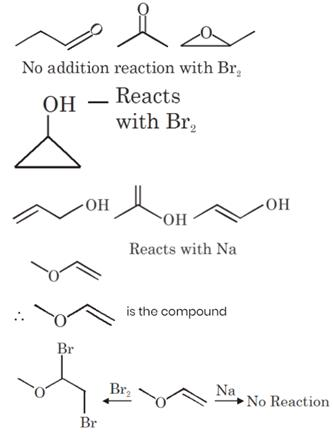VD × 2 = MM
= 29 × 2 = 58
∴ 58 g of ether combines with 2 × 80g Br2
∴  2.9 g of ether will combines with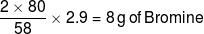Y = 9 and X = 8
Y – X = 9 – 8 = 1

*Answer can only contain numeric values
QUESTION: 24

How many of the following reagent/s will liberate at least one oxide of nitrogen as a product ?
(i) Ag + conc. HNO3
(ii) Sn + dil., HNO3 (20%)
(iii) Cu + conc. HNO3
(iv) C + conc. HNO3
(v) Zn + conc, HNO3
(vi) Zn + dil. HNO3 (20%)
(vii) P4 + conc. HNO3
(viii) S8 + conc. HNO3
(ix) Cu + dil. HNO3 (20%)

Solution:

(i) give NO2        (ii) NH4NO3
(iii) gives NO2     (iv) Give NO2
(v) give NO2          (vi) Given N2O
(vii) Gives NO2     (viii) Gives NO2
(ix) Gives NO

*Answer can only contain numeric values
QUESTION: 25

In white phosphorous (P4) if
x is total number of triangle
y is total number of plane of symmetry z is total number of P–P bond
then calculate value of (y + z)/x.

Solution:

x = 4
y = 6
z = 6
Ans. (3)